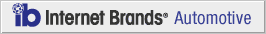# How to Compute the Accumulated Depreciation of a Vehicle

January 27, 2012

Knowing what the accumulated depreciation of a vehicle is going to be before you go into the car dealership is a great tool for making sure you get the right car. You will also want to know what this number is when you currently own a car and want to sell it or trade it in. When you purchase a car there is a certain amount of depreciation that happens the moment you drive it off the lot. This value can vary wildly according to make, model, mileage, condition, and added luxury comforts. If you do not know how to compute the accumulated depreciation, or figure out a used car value, here are the steps to take.

#### What You Need

• Calculator
• Vehicle Identification Number
• Computer

#### Step 1: Find Total Cost of Vehicle

Look to find out what the total cost of the vehicle is that you are purchasing, or selling. If you are selling a used car, you will need to know the actual amount you bought the vehicle for. For example, if you purchased the car brand new for \$20,000, this is the amount you are going to be using.

#### Step 2: Find Salvage Estimate

After you know what the initial cost of the vehicle is, you need to know what the salvage estimate is going to be. You can locate this through your insurance company, or a website that has a value estimation calculator.

#### Step 3: Determine Estimated Useful Life

This calculation is determined by taking an estimate of how long the vehicle is actually going to be useful as reliable transportation. This does not mean for the life of it. For example, if you own the vehicle for 6 years, then this is your estimated value life.

#### Step 4: Figure out Accumulated Depreciation

Now you can use simple math to figure out the accumulated depreciation of your car. To do this you use a formula. The formula is cost minus the salvage value divided by the estimated useful life. If you have a car that was bought for \$20,000 and the salvage estimate is \$10,000, you are left with a \$10,000 figure. Divide this by the 6 years you own the vehicle and you are left with approximately \$1,700 of depreciation each year.

#### Step 5: Apply to Cost

Now that you know your car is going to lose at least \$1,700 per year, this will be applied to each successive year you own the vehicle. For example, after the first year, your car will only be valued at \$18,300. The second year another \$1,700 will be assessed, and so on, until you reach the total amount of time you own the car.

#### Step 6: Use Unit of Production Method

There is a second method to calculate the value, by using the amount of units manufactured that year. This method only requires you know the cost and salvage estimate along with the units produced. To find this last number you will need to do some searching on the Internet for units produced in that year. If you car cost \$20,000 and the salvage cost is \$2,000, then you would be left with \$18,000. Divide this by the total number produced. If the total was 90,000 units, then the end number would be \$0.20. If you have 20,000 miles on your vehicle, multiply it by the \$.20 to find the yearly depreciation value of \$4,000 per year.

COPYRIGHT 1999-2020 MH Sub I, LLC dba CarsDirect.com